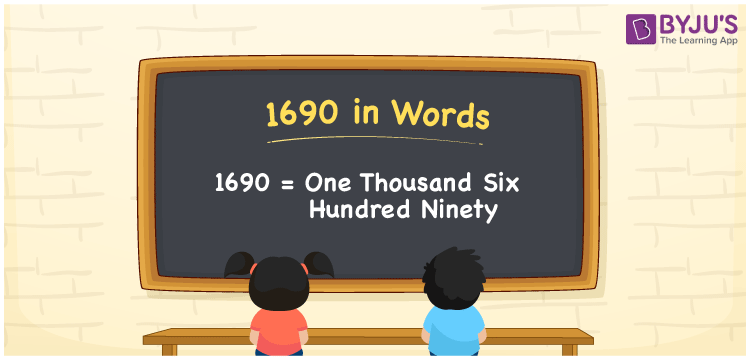# 1690 in words

1690 in words is written as One Thousand Six Hundred and Ninety. 1690 represents the count or value. The article on Counting Numbers can give you an idea about count or counting. The number 1690 is used in expressions that relate to money, distance, length, year and others. Let us consider an example for 1690. “The Planet Uranus was first sighted and recorded as a star in the year One Thousand Six Hundred and Ninety .” Another example, “A total of One Thousand Six Hundred and Ninety items were sold in a shop today.”

 1690 in words One Thousand Six Hundred and Ninety One Thousand Six Hundred and Ninety in Numbers 1690

## 1690 in English Words## How to Write 1690 in Words?

We can convert 1690 to words using a place value chart. The number 1690 has 4 digits, so let’s make a chart that shows the place value up to 4 digits.

 Thousands Hundreds Tens Ones 1 6 9 0

Thus, we can write the expanded form as:

1 × Thousand + 6 × Hundred + 9 × Ten + 0 × One

= 1 × 1000 + 6 × 100 + 9 × 10 + 0 × 1

= 1690

= One Thousand Six Hundred and Ninety.

1690 is the natural number that is succeeded by 1689 and preceded by 1691.

1690 in words – One Thousand Six Hundred and Ninety.

Is 1690 an odd number? – No.

Is 1690 an even number? – Yes.

Is 1690 a perfect square number? – No.

Is 1690 a perfect cube number? – No.

Is 1690 a prime number? – No.

Is 1690 a composite number? – Yes.

## Solved Example

1. Write the number 1690 in expanded form

Solution: 1 × 1000 + 6 × 100 + 9 × 10 + 0 × 1

We can write 1690 = 1000 + 600 + 90 + 0

= 1 × 1000 + 6 × 100 + 9 × 10 + 0 × 1

## Frequently Asked Questions on 1690 in words

Q1

### How to write 1690 in words?

1690 in words is written as One Thousand Six Hundred and Ninety.
Q2

### State if True or False. 1690 is divisible by 2?

True. 1690 is divisible by 2.
Q3

### Is 1690 a perfect cube number?

No. 1690 is not a perfect cube number.